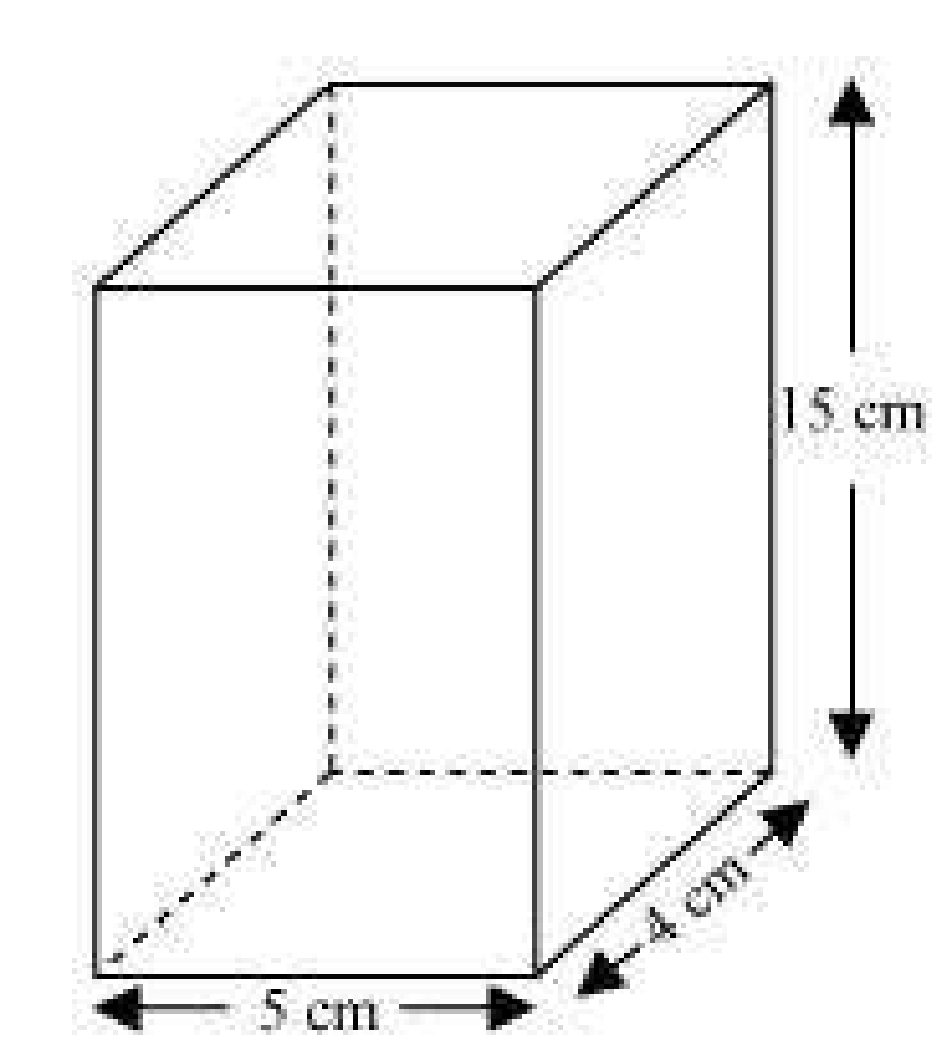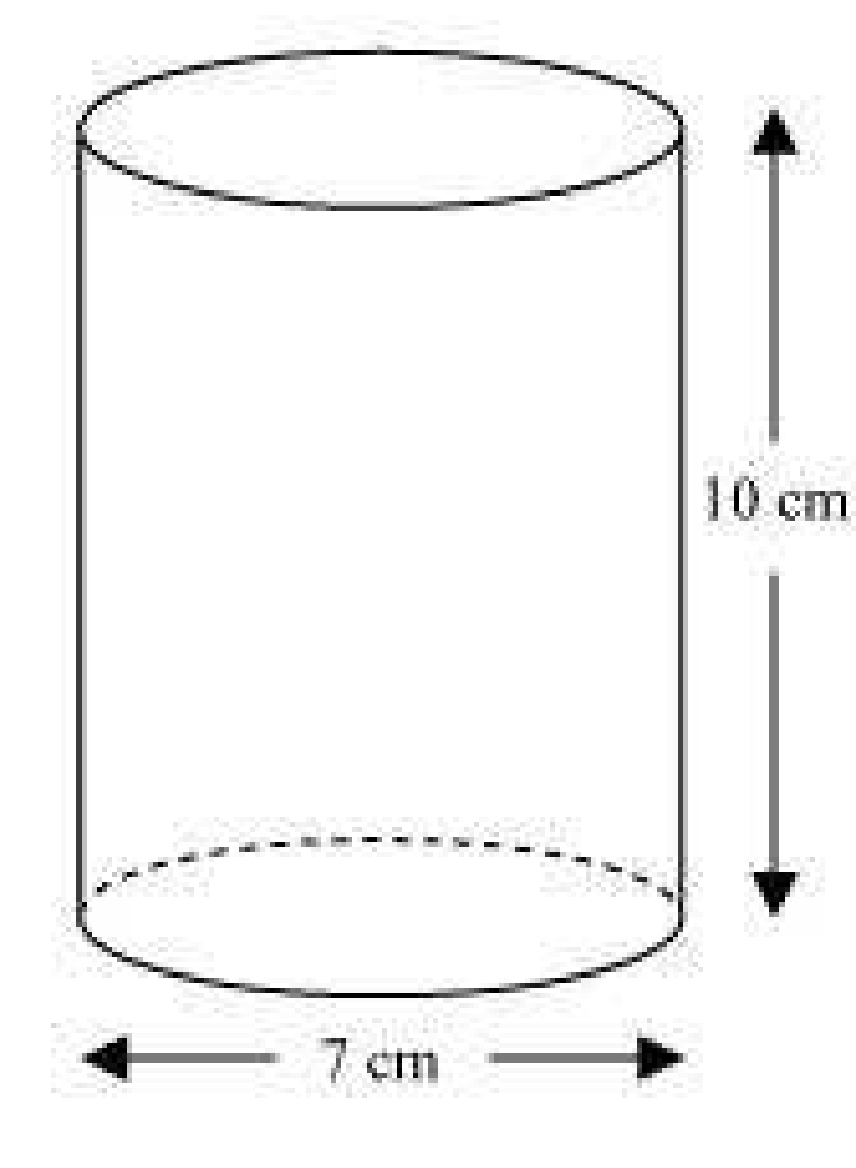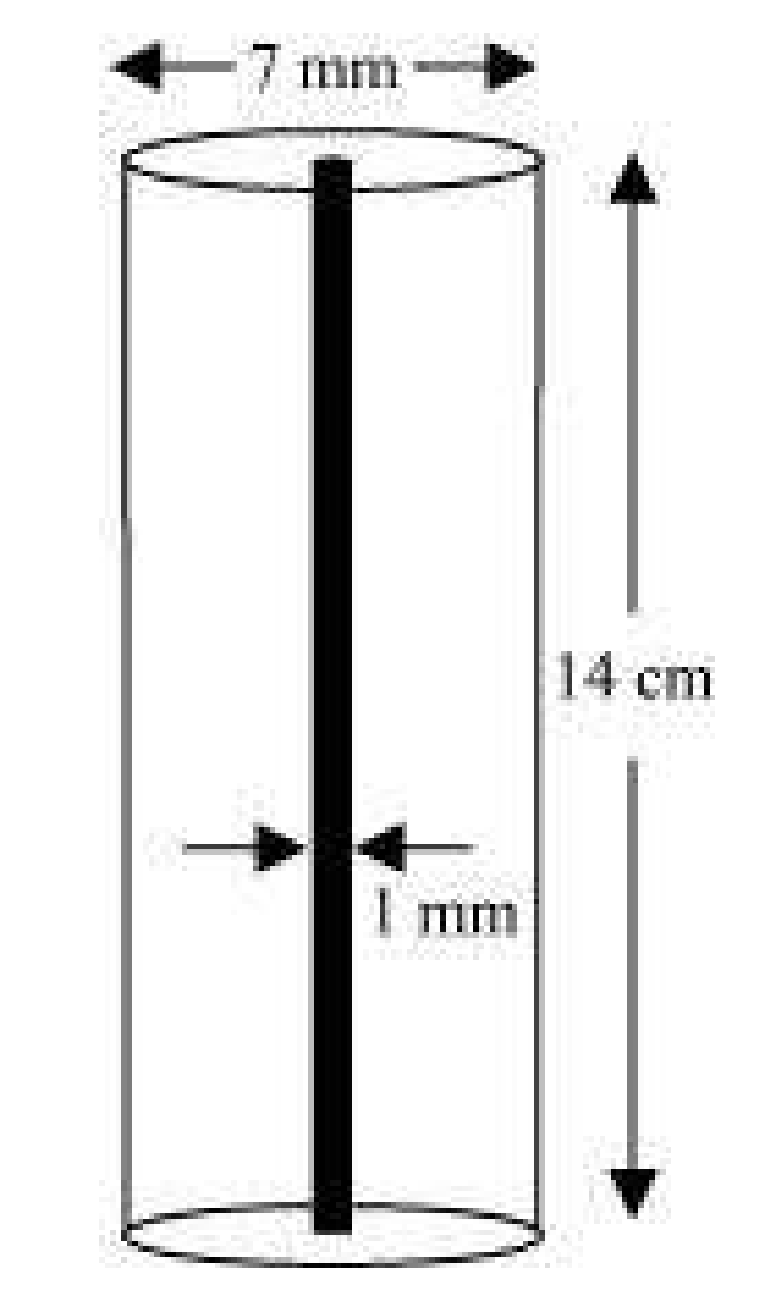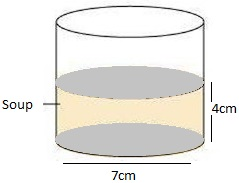2. ## The circumference of the base of cylindrical vessel is 132cm and its height is 25cm. How many litres of water can it hold? (1000 cm3= 1L) (Assume π = 22/7) Q.1

Circumference of the base of cylindrical vessel = 132 cm Height of vessel, h = 25 cm Let r be the radius of the cylindrical vessel. Step 1: Find the radius of vessel We know that, circumference of base = 2πr, so 2πr = 132 (given) r = (132/(2 π)) r = 66×7/22 = 21 Radius is 21 cm Step 2: Find the voluRead more

Circumference of the base of cylindrical vessel = 132 cm

Height of vessel, h = 25 cm

Let r be the radius of the cylindrical vessel.

Step 1: Find the radius of vessel

We know that, circumference of base = 2πr, so

2πr = 132 (given)

r = (132/(2 π))

r = 66×7/22 = 21

Step 2: Find the volume of vessel

Formula: Volume of cylindrical vessel = πr2h

= (22/7)×212×25

= 34650

Therefore, volume is 34650 cm3

Since, 1000 cm3 = 1L

So, Volume = 34650/1000 L= 34.65L

Therefore, vessel can hold 34.65 litres of water.

See less
• 0
• Share
3. ## The inner diameter of a cylindrical wooden pipe is 24cm and its outer diameter is 28 cm. The length of the pipe is 35cm.Find the mass of the pipe, if 1cm3 of wood has a mass of 0.6g. (Assume π = 22/7) Q.2

Inner radius of cylindrical pipe, say r1 = diameter1/ 2 = 24/2 cm = 12cm Outer radius of cylindrical pipe, say r2 = diameter2/ 2 = 28/2 cm = 14 cm Height of pipe, h = Length of pipe = 35cm Now, the Volume of pipe = π(r22-r12)h cm3 Substitute the values. Volume of pipe = 110×52 cm3 = 5720 cm3 Since,Read more

Inner radius of cylindrical pipe, say r1 = diameter1/ 2 = 24/2 cm = 12cm

Outer radius of cylindrical pipe, say r2 = diameter2/ 2 = 28/2 cm = 14 cm

Height of pipe, h = Length of pipe = 35cm

Now, the Volume of pipe = π(r22-r12)h cm3

Substitute the values.

Volume of pipe = 110×52 cm3 = 5720 cm3

Since, Mass of 1 cm3 wood = 0.6 g

Mass of 5720 cm3 wood = (5720×0.6) g = 3432 g or 3.432 kg. Answer!

See less
• 0
• Share
4. ## A soft drink is available in two packs – (i) a tin can with a rectangular base of length 5cm and width 4cm, having a height of 15 cm and (ii) a plastic cylinder with circular base of diameter 7cm and height 10cm. Which container has greater capacity and by how much? (Assume π=22/7) Q.3

tin can will be cuboidal in shape Dimensions of tin can are Length, l = 5 cm Breadth, b = 4 cm Height, h = 15 cm Capacity of tin can = l×b×h= (5×4×15) cm3 = 300 cm3 Plastic cylinder will be cylindrical in shape. Dimensions of plastic can are: Radius of circular end of plastic cylinder, r = 3.5cm HeiRead more

1. tin can will be cuboidal in shapeDimensions of tin can are

Length, l = 5 cm

Height, h = 15 cm

Capacity of tin can = l×b×h= (5×4×15) cm3 = 300 cm3

1. Plastic cylinder will be cylindrical in shape.Dimensions of plastic can are:

Radius of circular end of plastic cylinder, r = 3.5cm

Height , H = 10 cm

Capacity of plastic cylinder = πr2H

Capacity of plastic cylinder = (22/7)×(3.5)2×10 = 385

Capacity of plastic cylinder is 385 cm3

From results of (i) and (ii), plastic cylinder has more capacity.

Difference in capacity = (385-300) cm3 = 85cm3

See less
• 0
• Share
5. ## If the lateral surface of a cylinder is 94.2cm2 and its height is 5cm, then find (i) radius of its base (ii) its volume.[Use π= 3.14] Q.4

CSA of cylinder = 94.2 cm2 Height of cylinder, h = 5cm (i) Let radius of cylinder be r. Using CSA of cylinder, we get 2πrh = 94.2 2×3.14×r×5 = 94.2 r = 3 radius is 3 cm (ii) Volume of cylinder Formula for volume of cylinder = πr2h Now, πr2h = (3.14×(3)2×5) (using value of r from (i)) = 141.3 VolumeRead more

CSA of cylinder = 94.2 cm2

Height of cylinder, h = 5cm

(i) Let radius of cylinder be r.

Using CSA of cylinder, we get

2πrh = 94.2

2×3.14×r×5 = 94.2

r = 3

(ii) Volume of cylinder

Formula for volume of cylinder = πr2h

Now, πr2h = (3.14×(3)2×5) (using value of r from (i))

= 141.3

Volume is 141.3 cm3

See less
• 0
• Share
6. ## It costs Rs 2200 to paint the inner curved surface of a cylindrical vessel 10m deep. If the cost of painting is at the rate of Rs 20 per m2, find (i) inner curved surface area of the vessel (ii) radius of the base (iii) capacity of the vessel (Assume π = 22/7) Q.5

(i) Rs 20 is the cost of painting 1 m2 area. Rs 1 is the cost to paint 1/20 m2 area So, Rs 2200 is the cost of painting = (1/20×2200) m2 = 110 m2 area The inner surface area of the vessel is 110m2. (ii) Radius of the base of the vessel, let us say r. Height (h) = 10 m and Surface area formula = 2πrhRead more

(i) Rs 20 is the cost of painting 1 m2 area.

Rs 1 is the cost to paint 1/20 m2 area

So, Rs 2200 is the cost of painting = (1/20×2200) m2

= 110 m2 area

The inner surface area of the vessel is 110m2.

(ii) Radius of the base of the vessel, let us say r.

Height (h) = 10 m and

Surface area formula = 2πrh

Using result of (i)

2πrh = 110 m2

2×22/7×r×10 = 110

r = 1.75

(iii) Volume of vessel formula = πr2h

Here r = 1.75 and h = 10

Volume = (22/7)×(1.75)2× 10 = 96.25

Volume of vessel is 96.25 m3

Therefore, the capacity of the vessel is 96.25 m3 or 96250 litres.

See less
• 0
• Share
7. ## The capacity of a closed cylindrical vessel of height 1m is15.4 liters. How many square meters of metal sheet would be needed to make it? (Assume π = 22/7) Q.6

Height of cylindrical vessel, h = 1 m Capacity of cylindrical vessel = 15.4 litres = 0.0154 m3 Let r be the radius of the circular end. Now, Capacity of cylindrical vessel = (22/7)×r2×1 = 0.0154 After simplifying, we get, r = 0.07 m Again, total surface area of vessel = 2πr(r+h) = 2×22/7×0.07×(0.07+Read more

Height of cylindrical vessel, h = 1 m

Capacity of cylindrical vessel = 15.4 litres = 0.0154 m3

Let r be the radius of the circular end.

Now,

Capacity of cylindrical vessel = (22/7)×r2×1 = 0.0154

After simplifying, we get, r = 0.07 m

Again, total surface area of vessel = 2πr(r+h)

= 2×22/7×0.07×(0.07+1)

= 0.44×1.07

= 0.4708

Total surface area of vessel is 0.4708 m2

Therefore, 0.4708 m2 of the metal sheet would be required to make the cylindrical vessel.

See less
• 0
• Share
8. ## A lead pencil consists of a cylinder of wood with solid cylinder of graphite filled in the interior. The diameter of the pencil is 7 mm and the diameter of the is 1 mm. If the length of the pencil is 14 cm, find the volume of the wood and that of the graphite. (Assume π = 22/7) Q.7

Radius of pencil, r1 = 7/2 mm = 0.7/2 cm = 0.35 cm Radius of graphite, r2 = 1/2 mm = 0.1/2 cm = 0.05 cm Height of pencil, h = 14 cm Formula to find, volume of wood in pencil = (r12-r22)h cubic units Substitute the values, we have = [(22/7)×(0.352-0.052)×14] = 44×0.12 = 5.28 This implies, volume of wRead moreRadius of pencil, r1 = 7/2 mm = 0.7/2 cm = 0.35 cm

Radius of graphite, r2 = 1/2 mm = 0.1/2 cm = 0.05 cm

Height of pencil, h = 14 cm

Formula to find, volume of wood in pencil = (r12-r22)h cubic units

Substitute the values, we have

= [(22/7)×(0.352-0.052)×14]

= 44×0.12

= 5.28

This implies, volume of wood in pencil = 5.28 cm3

Again,

Volume of graphite = r22h cubic units

Substitute the values, we have

= (22/7)×0.052×14

= 44×0.0025

= 0.11

So, the volume of graphite is 0.11 cm3.

See less
• 0
• Share
9. ## A patient in a hospital is given soup daily in a cylindrical bowl of diameter 7cm. If the bowl is filled with soup to a height of 4cm, how much soup does the hospital have to prepare daily to serve 250 patients? (Assume π = 22/7) Q.8

Diameter of cylindrical bowl = 7 cm Radius of cylindrical bowl, r = 7/2 cm = 3.5 cm Bowl is filled with soup to a height of4cm, so h = 4 cm Volume of soup in one bowl= πr2h (22/7)×3.52×4 = 154 Volume of soup in one bowl is 154 cm3 Therefore, Volume of soup given to 250 patients = (250×154) cm3= 3850Read more

Diameter of cylindrical bowl = 7 cm

Radius of cylindrical bowl, r = 7/2 cm = 3.5 cm

Bowl is filled with soup to a height of4cm, so h = 4 cmVolume of soup in one bowl= πr2h

(22/7)×3.52×4 = 154

Volume of soup in one bowl is 154 cm3

Therefore,

Volume of soup given to 250 patients = (250×154) cm3= 38500 cm3

See less
• 0
• Share
10. ## Triangles : The lengths of the diagonals of a rhombus are 24 cm and 32 cm. Calculate the length of the altitude of the rhombus.

Let ABCD be a rhombus with AC and BD as its diagonals. We know that the diagonals of a rhombus bisect each other at right angles. Let O be the intersecting point of both the diagonals. Let AC=24cm and BD=32cm OA=AC/2 OA= 24/2=12cm OB=BD/2 OB=32/2=16cm In rt.ΔAOB by Pythagoras theorem we have AB²=OA²Read more

Let ABCD be a rhombus with AC and BD as its diagonals.
We know that the diagonals of a rhombus bisect each other at right angles.
Let O be the intersecting point of both the diagonals.
Let AC=24cm and BD=32cm
OA=AC/2
OA= 24/2=12cm
OB=BD/2
OB=32/2=16cm
In rt.ΔAOB by Pythagoras theorem we have
AB²=OA²+OB²
=(12)²+(16)²
=144+256
=400
AB=20cm
Hence, each side of the rhombus is of length 20cm
Area of rhombus=1/2*AC*BD
=1/2*24*32
=12*32
Area of rhombus=base*altitude
12*32=20*h
19.2cm=h

See less
• 15
• Share